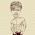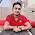# Fibonacci Series with C Program

The Fibonacci Sequence is the series of numbers:
0, 1, 1, 2, 3, 5, 8, 13, 21, 34, ...
The next number is found by adding up the two numbers before it.
• The 2 is found by adding the two numbers before it (1+1)
• Similarly, the 3 is found by adding the two numbers before it (1+2),
• And the 5 is (2+3),
• and so on!
Source: Math is Fun

#### C Program to Generate Fibonacci Series:

```#include <stdio.h>

int main()
{
int n, i, num1, num2, num;
num1 = 0;
num2 = 1;
printf("Enter number of terms: ");
scanf("%d",&n);
printf("First %d turms of Fibonacci series are:\n");
for(i=0;i<n;i++){
if(i<=1)
num = i;
else {
num = num1 + num2;
num1 = num2;
num2 = num;
}
printf("%d ",num);
}
return 0;
}
```
Share:

#### 4 comments :

1.Nice thinking to help others and develop yourself. All the best. Don't stop, carry on.

1.Thanks for your valuable comment. Your single comment has inspired me a lot. :)

2.This will be a huge help for those who're just starting to use c++ and code blocks. Accurate yet simple.

3.C++ Program to Generate Fibonacci series

Fibonacci Series is in the form of 0, 1, 1, 2, 3, 5, 8, 13, 21,...... To find this series we add two previous terms/digits and get next term/number.

Spam comments will be deleted. :)